# Uncertainty in Physical Measurements Module 4

Uncertainty Module 4 - UM Student Guide Jan. 23, 2017, 3:57 p.m.

## Repeated Measurements

In Modules 2 and Module 3 we considered a single measurement of some physical quantity. In each of the examples we discussed, repeating the measurement of the same object using the same instrument almost certainly would give the same result. So repeating these measurements doesn’t give us any added information about the value and uncertainty of the quantity being measured. In this Module we will think about cases where repeated measurements do not give the same value of the measurand, and you will measure the time for a piece of paper to fall to the floor.

We will begin by thinking about the following experimental apparatus.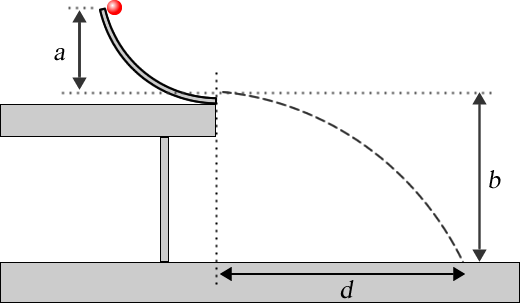#### Figure 1

A curved ramp is mounted on a table. You release a small ball from rest at the top of the ramp, it rolls down the ramp, and then travels along the dashed path. There is a special paper on the floor where the ball lands and when the ball strikes the paper it will leave a mark on it where it landed. We measure the horizontal distance d the ball travels when it hits the floor. It is hard for you to release the ball from exactly the same position each time, and the ramp and ball are not completely smooth so the ball bounces around a bit as it goes down the ramp. Therefore, if you repeat the measurement a few times, it is unlikely that the ball landed in exactly the same place each time. Perhaps after 5 trials the paper looks like Figure 2. We call such measurements scattered or dispersed.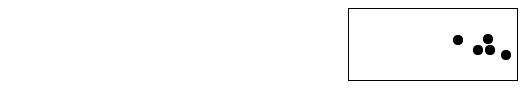#### Figure 2

It the absence of air resistance and for a very small ball, Newton’s Laws can be used to show that the theoretical value of d, dtheory is:

$$d_{\rm theory}=2\sqrt{ab}$$      (1)

The question, then, is does the data taken from Figure 2 match this theoretical prediction within the experimental uncertainty? Since more-or-less random factors have made the measurements of d dispersed, repeating the measurement will hopefully mean that the mean value of all the trials will give us a better estimate of the true value of the distance. We will return to this experiment later in this Module.

There are many other circumstances where more-or-less random factors cause the results of repeated measurements to not give the exact same result. You saw an example of this in Module 1 – Backgammon 101, when the results of rolling dice 36 times were different for different Teams. Similarly, the height of a person is related to factors like the nutrition of the person when a child, genetics, and other factors. In 1885 Francis Galton measured the heights of 928 adults in London, England. Figure 3 shows the results of his measurements. You can see that the shape is roughly a bell-shaped curve.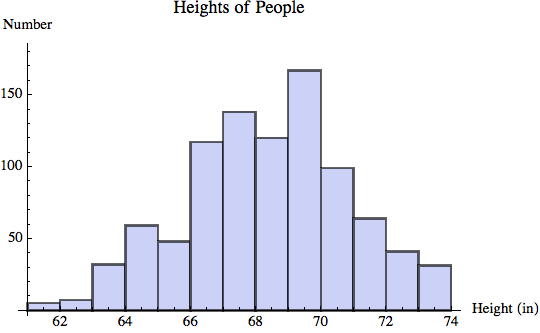#### Figure 3

Galton also invented an apparatus called a quincunx or Galton board shown in Figure 4. In Part (a), a hopper filled with balls drops them one at a time onto a peg. There is a 50% chance the ball will bounce to the left and a 50% chance the ball will bounce to the right. There are two pegs under this peg, positioned so that for each there is a 50% chance that the ball that strikes it will bounce to the left and a 50% chance that it will bounce to the right. This continues until at the bottom, when the balls are collected into the bins. A possible result of running the apparatus is that the balls are distributed as shown in Part (b). This too is approximately a bell-shaped curve.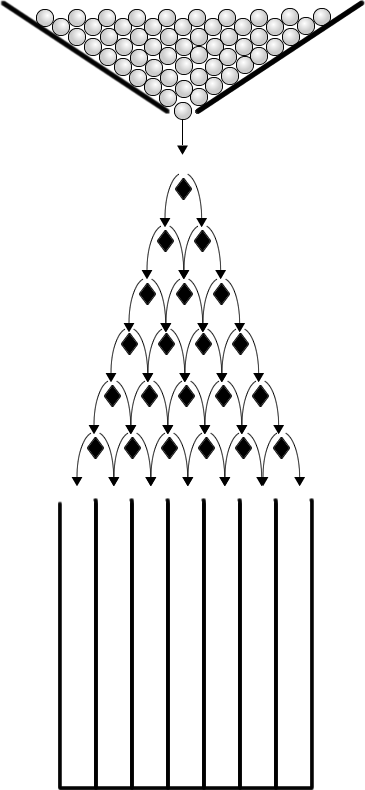(a)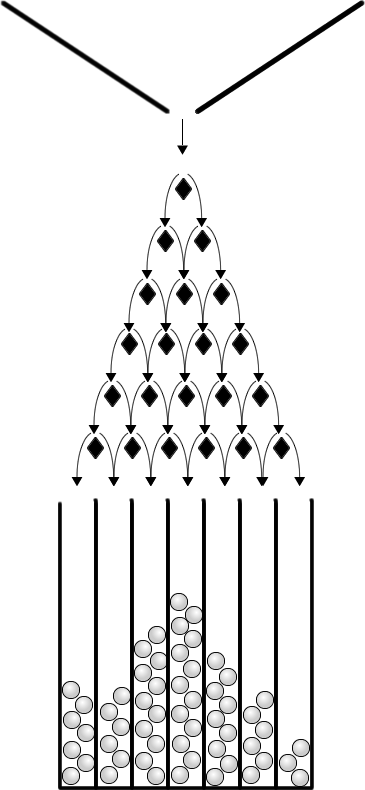(b)

Figure 4

Here is a link to a YouTube video of a real Galton board:  https://youtu.be/03tx4v0i7MA .

A final example of an approximately bell-shaped distribution is student marks on a test. Your mark on a test depends on your ability, how hard you have been studying, how you are feeling on the day you wrote the test, the degree that the questions on the test asked about things that you studied, and probably other factors. Figure 5 shows a histogram of marks for a 200-student Physics class on a recent Final Exam. It too is approximately bell-shaped. We will comment soon on the smooth curve that is also shown in the figure.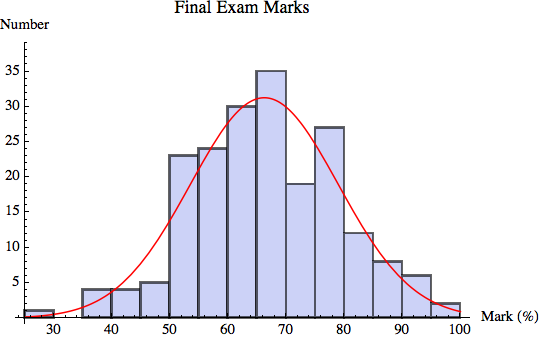#### Figure 5

Bell-shaped curves are often called Gaussian distributions because Carl Friedrich Gauss studied them extensively in the early 19th century. They occur so often that sometimes they are called normal distributions. We can write a formula for the amplitude n(x) of a bell-shaped curve for a variable x as:

$$n(x)=n_{\rm max}e^{-{(x-\mu)^2 \over 2\sigma^2}}$$      (2)

where nmax is the maximum value of n$$\mu$$ is the value x for which n(x) = nmax and $$\sigma$$ is the standard deviation. As you will soon see, this is the same standard deviation you learned about in Module 2 and Module 3.

The solid curve in Figure 5 shows the result of fitting the mark data to a Gaussian. The values of the fitted parameters are:

\begin{align*} n_{\rm max} &= 31.2 \pm 0.6 \\ \mu &= 66.2 \pm 0.3 \\ \sigma &= 12.8 \pm 0.3 \end{align*}     (3)

Now we will return to the experiment shown in Figure 1 with the data of Figure 2. We measure the horizontal position xi for each of the i trials with a ruler. For now we will ignore the uncertainty in the measurement by the ruler: instead we will concentrate on the spread of values that we see in Figure 2.

It is probably reasonable to model the data with a Gaussian probability distribution function. But there are at least two issues in forming this model.

Issue 1: As always, the total area under the pdf must equal to 1. But the area A under a Gaussian can be shown from integral calculus to be:

$$A=\sqrt{2 \pi} \times n_{\rm max} \times \sigma$$        (4)

Therefore for a probability distribution function nmax must be related to $$\sigma$$ by:

$$\begin{eqnarray*} n_{\rm max}={1 \over \sqrt{2\pi} \sigma} \end{eqnarray*}$$      (5)

Figure 6 shows two Gaussian pdfs, both with total areas equal to 1. Both have values of $$\mu = 50$$ . The solid curve has $$\sigma=10$$ and the dashed curve has  ​$$\sigma=20$$​. We see that  ​$$\sigma$$​ is a measure of the width of the distribution.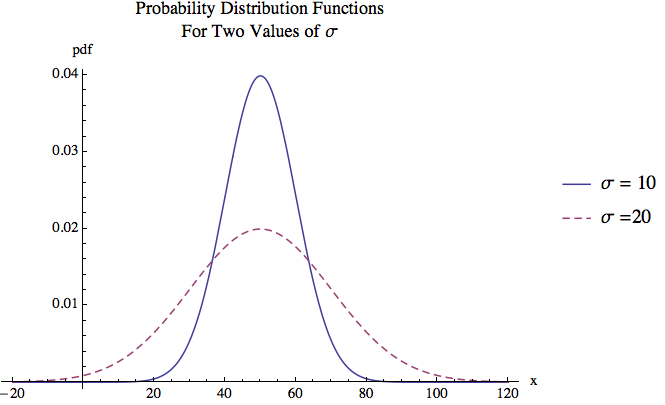#### Figure 6

Although we didn’t say so at the time, the standard deviation is also a measure of the width for the uniform and triangular probability distribution functions of Module 2 and Module 3. For both of these the half-width of the distribution is a and for the rectangular pdf

$$a=\sqrt{3}\sigma$$ while for the triangular pdf  ​$$a=\sqrt{6}\sigma$$​ . For Gaussians the half-width is not a measure of the width of the distribution, since it is always infinite.

Soon we will want to know that for each Gaussian in Figure 6, from integral calculus it can be shown that the area under the curve between $$\mu - \sigma$$ and  ​$$\mu + \sigma$$​ is 0.68.

##Question 1

Imagine that the data of the experiment in Figures 1 and 2 gives distances d between 0.62 and 0.73 m. True Gaussians, Eqn. 2, only approach zero asymptotically as $$x \rightarrow \pm \infty$$ . So if we use a Gaussian probability distribution function to describe the data of experiment of Figure 1, this says that there is a small but non-zero probability of getting a result of $$d=-432\ {\rm km}$$. Is this physically possible? What does this tell you about using a Gaussian pdf?

Issue 2: The second issue is more difficult. Although we may believe that the distribution of the values of x corresponds at least approximately to a Gaussian, unless we repeat the measurements an infinite number of times we can not know what that Gaussian is! With only a finite number of measurements, it is possible that the random factors that lead to a Gaussian distribution happened to work out so that most of the measured values of x were too high or too low, or perhaps they were spread out more widely or more narrowly than the actual distribution, or perhaps both.

To find the true mean of the data requires an infinite number of measurements:

$$\bar{x}_{\rm exact} \equiv \mu = \lim \limits_{N\to \infty}\left({1 \over N}\sum \limits_{i=1}^N x_i \right)$$     (6)

For a finite number of measurements we can only estimate the mean:

$$\bar{x}_{\rm est} = {1 \over N}\sum \limits_{i=1}^N x_i\ , \quad N \neq \infty$$       (7)

Since we can only estimate the mean, we can only estimate the variance and the standard deviation:

\begin{align*} var_{\rm est} & = {1 \over N-1} \sum_{i=1}^N(x_i-\bar{x}_{\rm est})^2 \\ \sigma_{\rm est} & = \sqrt{var_{\rm est}}=\sqrt{ {1 \over N-1} \sum_{i=1}^N(x_i-\bar{x}_{\rm est})^2} \end{align*}       (8)

Note that these equations are essentially identical to the equations for variance and standard deviation that we have seen in previous Modules except that they use the estimated mean since we cannot know the true value of the mean. Although the variance and standard deviation are just estimates, their interpretation is the same. For any individual measurement xi, the estimated uncertainty in the value of the measurand is:

$$u(x_i)=\sigma_{\rm est}$$      (9)

Note that this is not the uncertainty in the value of the estimated mean $$\bar{x}_{\rm est}$$: it is the uncertainty in each individual measurand xi. Above we stated that for a Gaussian pdf, the area under the curve between $$\mu - \sigma$$ and  ​$$\mu + \sigma$$​ is 0.68. Therefore it is reasonable to assume that the probability that for a single measurement xi the true value of $$\bar{x}$$ is within $$\sigma_{\rm est}$$ of xi is 0.68. Put another way, in the experiment of Figures 1 and 2 if modeling the pdf as a Gaussian is reasonable, then if you choose one of the measurements of the distance xi at random, there is a 68% chance that it is within one standard deviation of the true value of the position.

Since this uncertainty arises from the scatter of values due to various random effects, this type of uncertainty is often called statistical.

It can be shown that if a measurement is repeated N times, the estimated uncertainty in the standard deviation, $$u(\sigma_{\rm est})$$, is given by:

$$\begin{eqnarray*} {u(\sigma_{\rm est}) \over \sigma_{\rm est}}={1 \over \sqrt{2(N-1)}} \end{eqnarray*}$$       (10)

The quantity  $$u(\sigma_{\rm est})/\sigma_{\rm est}$$is called the fractional uncertainty in the estimated standard deviation. Multiplying this by 100 gives the percentage uncertainty.

##Activity 1

Imagine that you have measured the time for a pendulum to undergo five oscillations, t5, with a digital stopwatch. You repeat the measurements 4 times, and the data are:

 t5 (s) 7.53 7.38 7.47 7.43

You correctly calculate the estimated standard deviation of the measurements ​$$\sigma_{\rm est}$$​ , and the display on your calculator reads 0.0634429. From Eqn. 10, calculate the value of ​$$u(\sigma_{\rm est})$$​ . Express your answer to the same number of significant figures as the given value of ​$$\sigma_{\rm est}$$​ .

We express this result by writing ​$$\sigma_{\rm est}\pm u(\sigma_{\rm est})$$​ , which is a compact way of saying that you think that the actual value of the standard deviation is probably between  ​$$\sigma_{\rm est} - u(\sigma_{\rm est})$$​ and ​$$\sigma_{\rm est} + u(\sigma_{\rm est})$$​ .

1. Write down ​$$\sigma_{\rm est} - u(\sigma_{\rm est})$$​ and ​$$\sigma_{\rm est} + u(\sigma_{\rm est})$$​ to one significant figure.

2. Write down ​$$\sigma_{\rm est} - u(\sigma_{\rm est})$$​ and ​$$\sigma_{\rm est} + u(\sigma_{\rm est})$$​ to two significant figures.

3. Write down ​$$\sigma_{\rm est} - u(\sigma_{\rm est})$$​ and ​$$\sigma_{\rm est} + u(\sigma_{\rm est})$$​ to three significant figures.

Considering the facts that the ranges of values you specified in all three cases are only expressions of the range where you think the actual value probably lies, and that the value of the standard deviation itself is only an estimate, do you think there is any meaningful information to communicate to others by giving the range of values to two or three significant figures instead of just to one significant figure?

What can you conclude about the number of digits in the value of the estimated standard deviation that are actually significant? Is there any real meaning to the number 3 in the thousandths place, or the number 4 in the ten-thousandths place?You may have seen other definitions and ways of dealing with significant figures elsewhere. For experimentally determined quantities, such as  ​$$\sigma_{\rm est}$$​ in Activity 1, those definitions and properties are not appropriate! The number of significant figures in an experimentally determined value is defined by its uncertainty,  ​$$u(\sigma_{\rm est})$$​ in this case.

## Propagation of Uncertainties

Say we have measured some quantity x with uncertainty u(x) and a quantity y with uncertainty u(y) and wish to combine them to get a value z with uncertainty u(z). As we discussed in Module 2, we need the combination to preserve the probabilities associated with the uncertainties in x and y. We will consider a number of ways of combining the quantities. Although this Module has been discussing statistical uncertainties, this section applies to all uncertainties, including the ones you learned about in Module 2 and Module 3.

As discussed in Module 2 and Module 3, if z = x + y or z = xy then the uncertainties are combined in quadrature:

$$u(z)=\sqrt{u(x)^2 + u(y)^2}$$       (11)

#### Multiplication or Division

If z = xy or z = x/y then the fractional uncertainties are combined in quadrature:

$$\begin{eqnarray*} {u(z) \over |z|} = \sqrt{\left({u(x) \over x}\right)^2 + \left({u(y) \over y}\right)^2} \end{eqnarray*}$$​       (12)

#### Multiplication by a Constant

If z = ax, where a is a constant known to a large number of significant figures, then the uncertainty in z is given by Eqn. 12 with the uncertainty in a, u(a) = 0. So:

$$u(z) = |a| u(x)$$      (13)

#### Raising to a Power

If z = xn then:

$$u(z) = n x^{(n-1)}u(x)$$      (14)

which can also be written in terms of the fractional uncertainties:

$$\begin{eqnarray*} {u(z) \over z} = n{u(x) \over x} \end{eqnarray*}$$     (15)Say you are squaring x, so $$z = x^2 = x \times x$$. You may be tempted to use Eqn 12 for multiplication and division, but this is incorrect: Eqn 12 assumes that the uncertainties in the quantities x and y are independent of each other. Here there is only one quantity, x.

Be sure to remember that in call cases u(z) defines the significant figures in u.

#### The General Case

In general z is some function of x and y, z = f(x, y). The uncertainty in z requires knowing about partial derivatives. If you don’t know about these yet, you may skip this sub-section and go to the questions. Nonetheless:

$$u(z)=\sqrt{\left[ {\partial f(x,y) \over \partial x}u(x)\right]^2+\left[ {\partial f(x,y) \over \partial y}u(y)\right]^2}$$      (16)

Eqns. 11 – 15 are just applications of Eqn. 16 for various functions.

##Question 2

Eqn. 14 may look familiar to you. What does it look like? Hint: try writing u(z) as dz and u(x) as dx.Question 2

You measure a quantity to be $$3 \pm 1$$ and another quantity to be $$70 \pm 2$$ . What is the uncertainty in the sum to one significant figure? Does the uncertainty in the value of 3 have any effect on the uncertainty in the sum to one significant figure? Write down the sum $$\pm$$ its uncertainty to the correct number of significant figures. Remember from Activity 1 that the uncertainty only has one or at the very most two digits that really are significant, and that the uncertainty determines the number of digits in the value that are significant.

In 1998 Andrew Wakefield and collaborators published a fraudulent study in the medical journal The Lancet that claimed to show a link between autism spectrum disorder (ASD) and the combined measles, mumps, and rubella (MMR) vaccine. Since then many studies have been done claiming to show that there is no link between ASD and MMR. In 2015 Anjali Jain et al. published a new study in The Journal of the American Medical Association that involved a large sample of children in the U.S. You can see Jain’s paper at: http://jama.jamanetwork.com/article.aspx?articleid=2275444.

Here is a fragment of their data, that of four-year olds without an older sibling with ASD.

 MMR Vaccination Status Number of ASD Cases Sample Size 1 dose 395 79 691 Unvaccinated 65 11 957

Here are the data for four-year olds with and without an older sibling with ASD, regardless of whether or not they had received the MMR vaccine.

 Older Sibling with ASD Number of ASD Cases Sample Size No 460 91 648 Yes 89 1 878

As you will learn in Module 6, for numbers that are the result of counting, such as the 395 four-year olds without an older sibling with ASD, who had been vaccinated, and who had been diagnosed with ASD, a good first guess of the standard uncertainty in the number is the square root of that number.

##Question4

For four-year olds without an older sibling with ASD, does the Jain study indicate a correlation between MMR vaccination and ASD? For simplicity, assume that the uncertainties in the sample sizes are negligible. A good way to find the answer to this question is to:

1. Calculate the rate of four-year olds with ASD who had been vaccinated, including its uncertainty. The rate is the number of cases divided by the sample size. Your results will be easier to read if you express the value in scientific form, i.e as $$m \times 10^n$$  where n is an integer and $$1 \le |m| < 10$$ . Then express the uncertainty using the same notation but with the same value of n. For example: $$123.4 \pm 9.8 = (1.234 \pm 0.098) \times 10^2$$ .

2. Calculate the rate of four-year olds with ASD who had not been vaccinated, including its uncertainty.

3. Calculate the difference in these two rates, including the total uncertainty.

##Question 5

For four-year olds, does the study indicate a correlation with whether or not they have an older sibling with ASD? For simplicity, assume that the uncertainties in the sample sizes are negligible.

## The Uncertainty in the Mean

We have seen that for N repeated measurements, x1, x2, … , xN, the statistical uncertainty in each individual measurand xi is the estimated standard deviation $$\sigma_{\rm est}$$. We now know enough to determine the uncertainty in the estimated mean, $$u(\bar{x}_{\rm est})$$. The estimated mean is given by:

\begin{align*} \bar{x}_{\rm est} & = {1 \over N} \sum_{i=1}^N x_i \\ & = { [x_1 \pm u(x_1)] + [x_2 \pm u(x_2)] + ... + [x_N \pm u(x_N)]\over N} \end{align*}     (17)

But the uncertainty in each individual measurement is the same, which we will call u(x): $$u(x) \equiv u(x_1) = u(x_2) = ... = u(x_N)$$. Combining all the uncertainties in the numerator in quadrature gives:

$$\begin{eqnarray*} \bar{x}_{\rm est} = { (x_1 + x_2 + ... + x_N) \pm \sqrt{N} u(x) \over N} \end{eqnarray*}$$      (18)

The numerator is divided by the constant N, so from Eqn. 13:

​$$\begin{eqnarray*} \bar{x}_{\rm est} = { (x_1 + x_2 + ... + x_N) \over N} \pm { u(x) \over \sqrt{N}} \end{eqnarray*}$$​     (19)

or:

$$\begin{eqnarray*} u(\bar{x}_{\rm est})={u(x) \over \sqrt{N} } \end{eqnarray*}$$     (20)

So repeating a measurement N times reduces the statistical uncertainty in the mean by a factor of $$1 / \sqrt{N}$$ times the uncertainty in each individual measurement. So repeating a measurement 4 times reduces the uncertainty by a factor of ½.

The fact that the uncertainty in the mean is less than the uncertainty in each individual measurement should not be a surprise: we repeat measurements precisely so that we increase our knowledge of the true value of what we are measuring, i.e. in order to reduce its uncertainty.

If we were actually doing the experiment of Figure 1, we finally could now determine if the measured value of the distance is within experimental uncertainties of the theoretical value of Eqn. 1.

##Question  6

For the made-up data of Activity 1, you correctly calculate the mean of the four measurements, $$\bar{t_5}$$. and your calculator reads 7.45250. Assume that the only significant uncertainty in each individual measurement of the time is the standard deviation. From Eqn. 20, what is the uncertainty in this mean value? Express your final result as $$\bar{t_5} \pm u(\bar{t_5})$$ . Be sure to only present digits that are significant.

##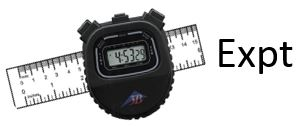Activity 2

Using the supplied digital stopwatch, try to start it and then stop it at exactly 2.00 s. Practice a few times before beginning to take the data. After practicing, repeat a few times. All members of the Team should do this, so you may end up with about 15 or 20 values. Just by looking at the data and without doing any calculations, choose a value of u such that most but not necessarily all measurements are between 2.00 – u and 2.00 + u.

##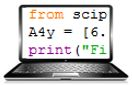Activity 3

It is a good idea to use Python to enter your data as you take it, just as you did for rolling dice in Module 1. It is probably an excellent idea to review how you used Python in that Module now.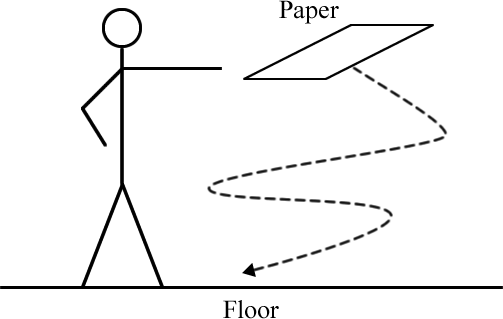You are supplied with a standard 8 ½ by 11 inch sheet of paper and a digital stopwatch. Hold the paper horizontally at shoulder height and release it. Measure the time t it takes the paper to reach the floor. Repeat for a total of 20 times, excluding trials where the paper strikes something as it falls.

Make a histogram of the results of your experiment by hand. You will need to decide how many bins to use in making the histogram. The decision is based somewhat on the scatter of values. Perhaps a good first guess is the number of datapoints divided by 2 rounded down to the nearest integer. You will also need to decide the values of t that separate the bins. In general, it is a good idea to make those values something easy for humans to read, such as 1.9, 2.0, 2.1, …, instead of something like 1.873, 1.973, 2.073 …

Is it reasonable to assume that the scatter of values of t can be described by a Gaussian probability distribution function? If not, can you think of another simple function that better describes the shape of the histogram? What is that shape, and why is it better?

What is the estimated statistical uncertainty in each measurement of t, i.e. the estimated standard deviation? The Python function to calculate standard deviations is std(). However, just as for the var() function you used in Module 1 to calculate the variances, by default the Python standard deviation function divides the N, not N – 1. So, just as for the variances, you will need to calculate std( data, ddof = 1).

In Activity 2 you estimated an uncertainty in the individual time measurements due to human reaction times, call it $$u_{\rm reaction}(t_i)$$. You have just found another uncertainty in the individual measurements, the one due do the random fluctuations in the times you measured for different trials; we will call this the statistical uncertainty ​$$u_{\rm statistical}(t_i)$$​ . It is reasonable to combine these two uncertainties in quadrature, the square root of the sum of the squares, to estimate the total uncertainty in each individual measurement.

Do the calculation of combining these two uncertainties. Remember from Question 3 that if one uncertainty is much smaller than the other, than when combining them in quadrature to only 1 or 2 significant figures the smaller value has negligible effect on the combination, and sometimes it is not even worth the effort of doing the calculation. Does the smaller of the uncertainties being combined here have a significant effect on the value of the combination?

Can you think of any other uncertainties, such as the reading uncertainty of a digital instrument or the accuracy of the stopwatch, which might have a significant effect on the total uncertainty in your measurements of ti? If so, calculate their effects.

Finally, what is estimated mean time for the paper to reach the floor, and what is the uncertainty in this time? Present your final result as $$\bar{t} \pm u(\bar{t})$$ .

##Question 7

Consider the following statement:

To properly study human reaction time, the methods and especially rough data analysis of Activity 2 are inadequate. But to determine the effect of reaction time on the measurement of the time for a piece of paper to fall to the floor in Activity 3, the methods used in Activity 2 are good enough.

Do you agree? Explain.

##Activity 4

This activity is not about the main topic of this Module, which is repeated measurements of the same quantity. Instead it is about uncertainties in measurements using analog instruments, which you learned about in Module 3, and propagation of uncertainties when the directly measured quantities are being divided, which you have learned about in this Module.

You are supplied some circular metal hoops of different sizes. For each hoop determine its diameter and its circumference with the supplied meter stick, and include the uncertainties in your determination of the diameter and circumference. A nice way to determine the circumference is to roll the hoop on the tabletop for exactly one revolution and measure how far it rolled.

Then, for each hoop calculate the circumference divided by the diameter, and the uncertainty in the ratio. Is the ratio the same value within the calculated uncertainties for all the hoops? Is there some theoretical value of the ratio? If so, what is it and are your measurements within uncertainties of this value? Also if so, if you repeated the measurement for a large number of hoops of different sizes, would you expect all of the calculated ratios to be within uncertainties of this value, and if not what fraction of them should be within uncertainties of the theoretical value?

## Looking Back

Modules 0 – 4 are the heart of our study of uncertainties in physical measurements. Although there is more to be learned in Modules 5 and 6, now is a good time to pause and look back at some of the things that we have learned so far about this topic.

A good experimentalist has brains in her/his fingertips, and has some intuitive sense of how precise their measurements are. For example, in the late 19th century physicist Lord Rayleigh (John Strutt) produced samples of nitrogen two different ways. One method isolated nitrogen from the air, and the other produced nitrogen from chemical sources. When he measured the density of the two samples, they were not quite the same. The density of the N2 from the sample from air was $$\rho_{\rm air} = 1.2572\ {\rm g/liter}$$ , while the density from the sample produced from chemical sources was  ​$$\rho_{\rm air} = 1.2505\ {\rm g/liter}$$​ The two values differ by only about 0.5%. Rayleigh was brilliant experimentalist, and his intuition told him that the two values were not the same within uncertainties, although he didn’t actually calculate those uncertainties. He presented these results in a talk in 1894. Chemist William Ramsay attended the talk, and together they later showed that the difference in densities was because the atmospheric nitrogen contained a heavy impurity. They identified the impurity as the previously unknown element Argon. They both received Nobel Prizes for this work, Rayleigh in physics and Ramsay in chemistry.

Uncertainty analysis like you have been learning about allows an experimentalist to quantify and communicate their intuition about the uncertainty in some experimentally determined quantity.

We have seen that uncertainty analysis involve a number of trade-offs and guesses. These include that:

• The choosing of a particular probability distribution function to characterize a measurement is almost always just an approximation or even just a wild guess.

• Sometimes, such as assigning the half-width a to a triangular pdf for analog measurements, there are no fixed rules and the experimentalist must do a trade-off between a large value, which may be too pessimistic, and a small value, which may be too optimistic.

Because of these trade-offs and guesses, we have seen that the uncertainty in some result is only known to one, or at most two, significant figures. We have also seen that the uncertainty is the definition of the number of significant figures in the value of the result.

Finally, the standard uncertainty u is always a statement that the actual value of the quantity being measured is probably within $$\pm u$$ of the stated value. So all uncertainties, whether due to reading an instrument, a manufacturer’s stated accuracy of the instrument, or a statistical one due to dispersed repeated measurements, are treated exactly the same way.

The standard uncertainty u is found the same way for all types of measurements, from the variance and the standard deviation $$\sigma$$.

$$\begin{eqnarray*} {\rm variance} = {1 \over N-1} \sum_{i = 1}^N (x_i - \bar{x})^2 \end{eqnarray*}$$      (21)

$$\sigma = \sqrt{\rm variance}$$      (22)

$$u = \sigma$$      (23)

When we combine two or more measurements to get a final result, we want to preserve the sense that the probability given by the uncertainty in the result is the same as the probabilities given by the uncertainties in the directly measured quantities. Since perhaps one of the directly measured quantities has a value that is too large and another is too small, it is possible for the uncertainties to cancel each other when they are combined. The correct procedure is to use a variation of Pythagoras’s theorem for right triangles, i.e. the square root of the sum of the squares. This procedure is called quadrature.

However, the values of the probability p expressed by the standard uncertainty using Eqns. 21 - 23 are somewhat different for different types of measurements. For digital measurements pdigital = 0.58, while for analog measurements panalog = 0.65, and for statistical uncertainties pstatistical = 0.68. You may be thinking that if we are trying to preserve the probabilities when we combine uncertainties in quadrature, surely the value of the probability should be the same for all types of measurements. In principle, one could do this by adjusting the relation between the uncertainty and the standard deviation, Eqn. 23. For example, if we wish the probability to be 0.65, the value for analog measurements, for all types of measurements we could define:

$$u_{\rm analog} = \sigma$$

$$u_{\rm digital} = {p_{\rm analog} \over p_{\rm digital}}\sigma = {0.65 \over 0.58} \sigma = 1.1 \sigma$$

$$u_{\rm statistical} = {p_{\rm analog} \over p_{\rm statistical}}\sigma = {0.65 \over 0.68} \sigma = 0.96 \sigma$$

However, to one significant figure the three values for the standard uncertainty actually have the same relationship to the standard deviation. We can reasonably conclude that this procedure is not worth the effort, and we just use Eqn. 23 for all types of measurements. Then when we combine uncertainties in different types of measurements we can ignore the insignificant differences in the values of the probabilities.

Finally, there is a common tendency to just write off the difference between some measured quantity and the expected or accepted value as due to human error. However, a moment’s reflection may convince that the phrase “human error” has no meaning whatsoever. Every measurement has an uncertainty, and in these Modules you have been learning about ways to quantify what that uncertainty is.

##Question 8

For the “experiment” of Figure 1, Eqn. 1 gives the distance as $$d_{\rm theory} = 2 \sqrt{ab}$$ . The acceleration due to gravity g does not appear in the equation. Is this reasonable? What about if the experiment were being done in a weightless environment such as the International Space Station, where g = 0?

## Summary of Names, Symbols, and Formulae

Scattered or dispersed values: when repeating a measurement gives a different value of the measurand.

Bell-shaped or Gaussian or normal distribution: a shape that can be described by:

​$$n(x)=n_{\rm max}e^{-{(x-\mu)^2 \over 2\sigma^2}}$$

Estimated mean: ​$$\begin{eqnarray*} \bar{x}_{\rm est} = {1 \over N}\sum \limits_{i=1}^N x_i\ \end{eqnarray*}$$

Estimated standard deviation: ​\begin{align*} \sigma_{\rm est} =\sqrt{ {1 \over N-1} \sum_{i=1}^N(x_i-\bar{x}_{\rm est})^2} \end{align*}

Significant figures: the number of digits in an experimentally determined quantity that have significance; found from the uncertainty in the value of that quantity.

Statistical uncertainty: the uncertainty that arises because the values of the measurand are scattered.

For a Gaussian probability distribution function:

The probability that the true value is within the statistical uncertainty  ​$$u(x)=\sigma_{\rm est}$$​ of an individual measured value xi is 0.68.

Fractional uncertainty: the uncertainty in a quantity divided by the value of that quantity.

Propagation of uncertainties:

Adding or subtracting two quantities: ​$$u(z)=\sqrt{u(x)^2 + u(y)^2}$$

Multiplying or dividing two quantities: ​$$\begin{eqnarray*} {u(z) \over |z|} = \sqrt{\left({u(x) \over x}\right)^2 + \left({u(y) \over y}\right)^2} \end{eqnarray*}$$

Multiplying a quantity by a constant a: ​$$u(z) = |a| u(x)$$

Raising a quantity to a power n: ​$$u(z) = n x^{(n-1)}u(x)$$

For a Gaussian probability distribution function, the statistical uncertainty in the estimated mean is:

$$\begin{eqnarray*} u(\bar{x}_{\rm est})={u(x) \over \sqrt{N} } \end{eqnarray*}$$

where u(x) is the uncertainty in each individual measurement and N is the number of times the measurement was repeated.

This Guide was written by David M. Harrison, Dept. of Physics, Univ. of Toronto, September 2013.

Modified by David M. Harrison, October 23, 2013; April 27, 2014.

Modified by David M. Harrison and Brian Wilson with suggestions from the Summer 2014 PHY131 instructors: May 31, 2014.

Question 6 and comments following Activity 1 added by David M. Harrison, November 1, 2014.

Jain data on autism and vaccination added. Questions 4 and 5 added. Subsequent questions re-numbered. David M. Harrison, August 20, 2015.